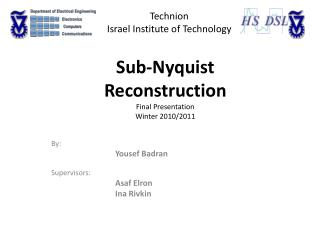DownloadDownload PresentationSub- Nyquist Reconstruction Final Presentation Winter 2010/2011

# Sub- Nyquist Reconstruction Final Presentation Winter 2010/2011

Download Presentation## Sub- Nyquist Reconstruction Final Presentation Winter 2010/2011

- - - - - - - - - - - - - - - - - - - - - - - - - - - E N D - - - - - - - - - - - - - - - - - - - - - - - - - - -
##### Presentation Transcript

1. Technion Israel Institute of Technology Sub-NyquistReconstructionFinal PresentationWinter 2010/2011 By: YousefBadran Supervisors: AsafElron Ina Rivkin

2. Project Overview • Part of the Modulated Wideband Converter project cluster. • A system for sub-Nyquist sampling of multiband signals. • Sub-module of the reconstruction block. • Implemented on a single NI FlexRio Virtex-5 SX95T FPGA device. • NI LabVIEW interface

3. Sub-NyquistXampling Project Block Diagram

4. Project Goals Reconstruction Algorithm outline: • Covert complex-valued sequences to real-valued sequences. • Estimate power spectral density. • Find band edges. COMPLEX FFT!!

5. Input and Output Input: Sequences z[n] representing spectrum slices. At most N sequences, one per slice. Spectral support slices s Problem: Spectral support and carrier frequencies of bands are unknown. A single slice may contain more than one band (at most N/2). A single band might be divided between two slices.

6. Input and Output • Output: • A sequence of vectors, one per spectral slice. • Each vector describes energy distribution in the slice; areas in the slice that • hold energy. • Requires high resolution vectors.

7. Welch’s Method for Power Estimation Algorithm • Divide the time signal into successive, overlapping blocks. • Form the periodogram for each block: • The Welsh estimate of the power spectral density is given by: • (K= # of blocks) • Contradiction: Maximize M for spectral resolution vs. Maximize K for better averaging results and greater spectral stability. • Typical choice:

8. Spectrum Quantization Final step: Define band location Find PSD threshold. Unite support regions that are closer than . Prune isolated regions with widths smaller than .

9. Implementation Demands Demands • 18-bit signed fixed-point representation (input). • 17 bit word length • 1 bit fraction length • Input rate of 20 MHz • Complex FFT • Implementation on a single FPGA • Use 30% of FPGA resources.

10. Project Block Diagram FIFO CTF module Low rate sequences Power Estimation Band detection output 20 MHZ

11. Testing Environment Host.vi

12. Host.vi (Write) Display Input Signal Host to FPGA FIFO(WRITE) 2048 elements Generate Sequence 1 MHz rate Parse data (64bit double to U32) Join numbers (U64bit) Real data MUX Img data 1024 Samples data Complex data Select

13. Host.vi (Read) Display Output Signal FPGA to Host FIFO (READ) 2048 elements Write to file 1024 Samples (U64 bit) DBL 1024 Samples (double) WELCH Display and compare results

14. FPGA Host to FPGA FIFO(READ) 2048 elements FPGA FIFO 1024 elements FPGA to Host FIFO(WRITE) 2048 elements FPGA FIFO 1024 elements Spectrum Quantization Real Split numbers (I32) FXP FXP Welch Power Spectral Estimation Img.

15. Welch PSD – Block Diagram Memory Read Addr. Write Addr. Counter Data out Data out Data in en Hamming Window Complex FFT Real data in Real data Real data Img. data in Img. data Img. data Out valid Shift reg

16. Quantization – Block Diagram

17. Welch’s Method for Power Estimation Comparison between MATLAB’s PWELCH, and my own: (M=256, R=128)

18. Results Complex FFT size = 1024.

19. Welch’s Method for Power Estimation Comparision between MATLAB’s PWELSH, and my own: (M=256, R=128)

20. Results Complex FFT size = 1024.

21. Project Workflow • Algorithm • MATLAB • Mathematical Tools • Find an alternative fixed • point FFT Solution • Find implementations of • required mathematical • operations in VHDL • Resources & • requirements • Understand theory and • reference implementation • Propose alternative • and equivalent calculation • Floating-point • representation • 18-bit fixed point • representation • Simulink FXP FFT • model • LabVIEW FPGA • Debug • Integrate system on • LabVIEW FPGA • NI-FlexRIO Virtex-5 • SX95T model. • Debug system • Timing considerations • Compare results • NI-LabVIEW • Creating a block diagram • Implement algorithm on • the host

22. Resource Estimation @ 40MHz, FFT size of 1024

23. Next Steps • Detect bands and isolate them. • Estimate carrier frequency per band. • 6 low rate sequences (20MHz) instead of 1.

24. Next Steps (Cont.) Low rate sequences (20MHz each) High rate Calculation 120 MHz FIFO 1024 elements FIFO 1024 elements FIFO 1024 elements FIFO 1024 elements Complex FFT & Hamming Window PSD & Band Detection FIFO 1024 elements FIFO 1024 elements FIFO 1024 elements MUX FIFO 1024 elements FIFO 1024 elements FIFO 1024 elements FIFO 1024 elements FIFO 1024 elements Serial FFT!

25. Thank you!